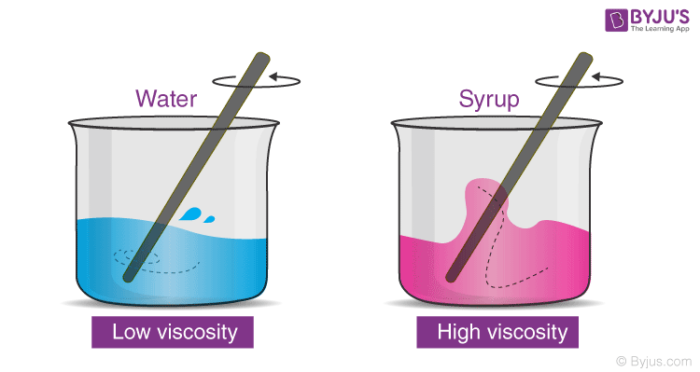# Coefficient of Viscosity

## What is Viscosity?

Viscosity is defined as the degree up to which a fluid resists the flow under an applied force; it is measured by the tangential friction force acting per unit area divided by the velocity gradient under conditions of streamline flow.

An important rheological measurement that is closely related to the resistance to flow is called viscosity.

The Viscosity of a fluid described as the resistance of liquid. For example liquids like water, alcohol, petrol and more flow freely and faster and have low viscosity than those that flow slowly like glycerin solution, honey, and oil. This is because of its physical property and is called viscosity. In simple words, it explains the fluid’s flow resistance.

## Recommended Videos## Viscosity Measurement

The viscosity is calculated in terms of the coefficient of viscosity. It is constant for a liquid and depends on its liquid’s nature. The Poiseuille’s method is formally used to estimate the coefficient of viscosity, in which the liquid flows through a tube at the different level of pressures.The coefficient of viscosity of fluids will be decreased as the temperature increases, while it is inverse in the case of gases. While the coefficient of viscosity of gases will increase with the increase in temperature. The increase in temperature for the fluid deliberate the bonds between molecules. These bonds are directly associated with the viscosity and finally, the coefficient is decreased.

An important question which people do not ask is why even measure Viscosity? The main reason behind this being, that viscosity data on a material gives manufacturers a chance to predict how the material will react and behave when consumers interact with it.

The most simplistic formula for measuring viscosity is:

$$\begin{array}{l}Viscosity = \frac{shear\ stress}{shear\ rate}\end{array}$$

## The Coefficient of Viscosity Formula

The force of friction between two layers of fluid having the area in square centimetre and separated by distance will have a velocity is given by:

$$\begin{array}{l}f\propto A \frac{dV}{dx}\end{array}$$

Or

$$\begin{array}{l}f = \eta \; A \; \frac{dV}{dx}\end{array}$$

Here, η is coefficient of viscosity and dV/dx is velocity gradient

## Viscosity Example

If  dx is 1cm,

A is 1cm2 and dv is 1cm/ s

Then,

$$\begin{array}{l}\small f = \eta\end{array}$$

The coefficient of viscosity is defined as the force of friction that is required to maintain a difference of velocity of 1cm/s between parallel layers of fluid. The unit is usually expressed in poise or centipoise. As we all know that viscosity is nothing but the measure of a substance’s resistance to the motion under an applied force The resulting unit is measured in centipoise which is the equivalent of 1 mPa (MilliPascal second)

The major industries where Viscosity measurements are used are in the food industry to improve the overall cost-effectiveness and maximise production efficiency. Besides the food industry Viscosity is also primarily used in other industries such as the Adhesives, Petroleum, Concrete, and Cosmetics industry.

For more information related to Viscosity, you can also check out the Dynamic Viscosity Formula, used for calculating internal resistance for fluids.